Corners and Junctions DetectionLab

Principal investigator: Robert Laganiere

Morphological Corner Detection

This operator uses a variant of the morphological closing operator, which we have called asymmetrical closing. It consists of the successive application of different morphological tranformations using different structuring elements. Each of these structuring elements used to probe the image under study is tuned to affect corners of different orientation and brightness.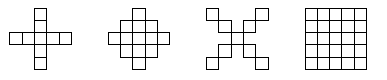The operator can be easily implemented with Intel OpenCV as follows:
```		cvDilate(src,tmp1,cross);
cvErode(tmp1,tmp2,lozeng);
cvDilate(src,dst,ex);
cvErode(dst,tmp1,square);
cvAbsDiff(tmp2,tmp1,dst);
if (threshold>0)
cvThreshold(dst,dst,threshold,255,CV_THRESH_BINARY);
```

### Publications:

Robert Laganičre,
Morphological Corner Detection ,
in Proc. International Conference in Computer Vision, pp. 280-285, Bombay, India, January 1998.
PDF [0.223 mb]

Robert Laganičre,
A Morphological Operator for Corner Detection ,
in Pattern Recognition, pp. 1643-1652, November 1998.
Online version

A modified version of this operator has been proposed in:

Shih, Frank Y.; Chuang, Chao-Fa; Gaddipati, Vijayalakshmi,
A modified regulated morphological corner detector ,
in Pattern Recognition Letters, pp. 931-937, April 2005.
Online version

### Source code

Available here. The file Comorph.h contains the operator's actual implementation that can be reused in other applications.

JUDOCA: JUnction Detection Operator based on Circumferential Anchors

Participant: Rimon Elias

This junction detection operator defines junctions as points where linear ridges in the gradient domain intersect. The radial lines that compose the junction are therefore identified by searching, in a circular neighborhood, for directional maxima of the intensity gradient. The proposed algorithm operates on two binary edge maps, the computational complexity of the detection process is then considerably reduced.

### Algorithm Outline:

1. Apply vertical and horizontal Gaussian derivative filters on image I.
2. Compute gradient magnitude and create two binary images from it:
1. The first one, B, is created from the imposition of a threshold, tB, on the gradient image.
2. The second one, B+, contains the points of B that are local maxima in the direction of the gradient.
3. For each point p in B, consider a circle of radius rho centered on this point and obtain the list of candidate points qi in B+ that lie on the circumference of this circle (see Figure 1). These so-called circumferential anchor (CA) points are the extremities of potential radial lines for the putative junction.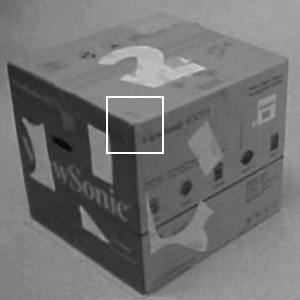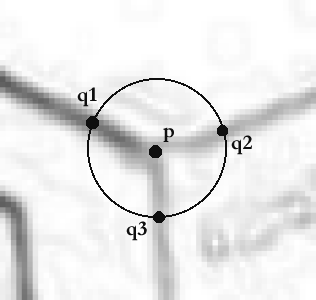(a) (b)
Figure 1: (a) One corner of a box that produces a Y-junction. (b) The junction at p with three circumferential anchors q1, q2 and q3 (superimposed on the gradient image).

4. For each CA point qi in the list, consider the set of all points located at a distance less than one pixel to the segment that joins the current CA point to the central point p. This set is used to determine if the corresponding putative junction radial line will be accepted (considering the points in B) and if yes, what the strength of this junction line would be (considering the points in B+), that is:
1. To be accepted as a junction line, a continuous path of B points joining the CA point and the central point p must exist. If not, then reject this radial line and repeat the scanning operation with the next CA point.
2. The strength, SJ(<p,qi>), of this junction radial line is defined as the sum of the squared distances from the B+ points in the currently considered set to the <p,qi> line segment. This strength is normalized by the length of this segment.
5. If the strength of this junction radial line is smaller than a predetermined threshold, sJ, then reject this radial line. Otherwise, <p,qi> becomes one of the branch of the putative junction at p.
6. If the number of branches found at p is less than 2, then there is no junction at this location. Otherwise, record the orientation of the accepted junction radial lines and set the strength, SJ(p), of this junction as being the minimum across all radial line strengths, i.e.:
SJ(p)= mini Sj(<p,qi>)

### Examples: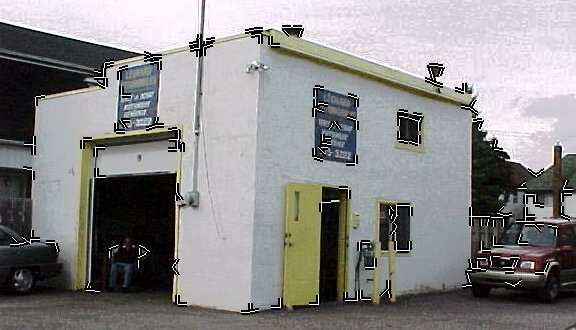(Click on image for better resolution)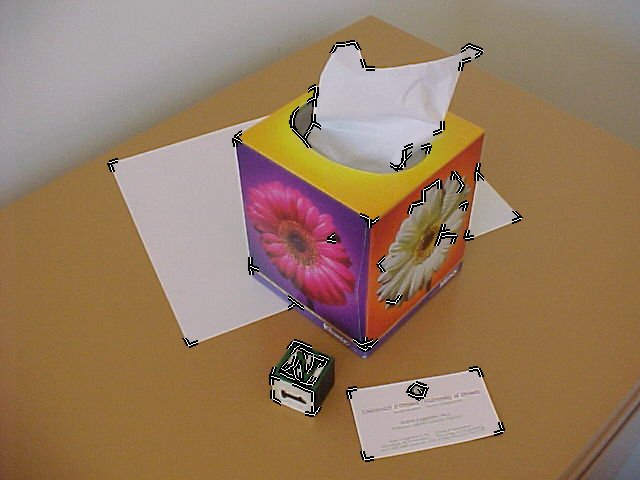(Click on image for better resolution)

### Publications:

Robert Laganičre, Rimon Elias
The Detection of Junction Features in Images ,
in Proc. International Conference on Acoustic, Speech and Signal processing, pp. 573-576, Montreal, Canada, May 2004.
PDF [0.223 mb]

### Source code

Available here.